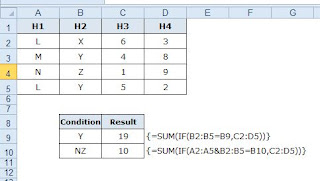## Posts

Showing posts from July, 2016

### Alternative for SUMIF and SUMIFSEver wondered while using "SUMIF" function, the sum range cannot be more than one column!!  This indeed is a big drawback while using a large data set where you need to sum matching certain conditions. I often end up using multiple sumif with "+" which makes the formula very long. So was thinking of some alternative that can make life easier. Thanks to the search engines these days, I did come across a workaround. Refer to the above image: In the above example, I have a table with 4 columns where H1 and H2 are identifiers. H3 and H4 is the data which needs to be summed up. Condition 1 (alternative for SUMIF): I need to sum values of H3 and H4 where the identifier H2 is "Y". The formula will be =SUM(IF(B2:B5=B9,C2:D5)) followed by Ctrl + Shift + Enter which will make it a array formula. The final formula will look like this:  {=SUM(IF(B2:B5=B9,C2:D5))} Condition 2  (alternative for SUMIFS) : I need to sum values of H3 and H4 where the